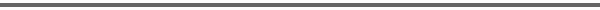Journal Home Page Complete Contentsof this Volume Previous Article Next Article Journal of Lie Theory 14 (2004), No. 2, 569--581 Copyright Heldermann Verlag 2004On the Principal Bundles over a Flag Manifold Hassan Azad Dept. of Mathematical Sciences, King Fahd University, Dhahran 31261, Saudi Arabia, hassanaz@kfupm.edu.sa Indranil Biswas School of Mathematics, Tata Institute of Fundamental Research, Homi Bhabha Road, Bombay 400005, India, indranil@math.tifr.res.in[Abstract-pdf] Let $P$ be a parabolic subgroup of a semisimple simply connected linear algebraic group $G$ over $\mathbb C$ and $\rho$ an irreducible homomorphism from $P$ to a complex reductive group $H$. We show that the associated principal $H$--bundle over $G/P$, associated for $\rho$ to the principal $P$--bundle defined by the quotient map $G\, \longrightarrow\, G/P$, is stable. We describe the Harder--Narasimhan reduction of the $G$--bundle over $G/P$ obtained using the composition $P\, \longrightarrow\, L(P)\, \longrightarrow\, G$, where $L(P)$ is the Levi factor of $P$. [FullText-pdf (172 KB)] for subscribers only.